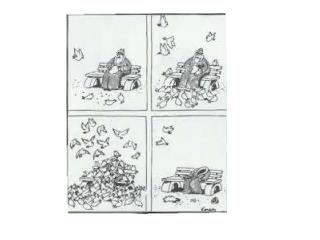DownloadDownload PresentationComplementary Angles

# Complementary Angles

Télécharger la présentation## Complementary Angles

- - - - - - - - - - - - - - - - - - - - - - - - - - - E N D - - - - - - - - - - - - - - - - - - - - - - - - - - -
##### Presentation Transcript

1. Complementary Angles Two or more angles whose measures add up to 90 degrees x 30

2. Supplementary Angles Two or more angles whose measures add up to 180 degrees 120 x

3. Vertical Angles Opposite Angles formed when two lines intersect. They are always equal! 140

4. Transversal A line that intersects two or more parallel lines. Corresponding Angles are congruent.

5. Find the missing angles.

6. My Transformation Buddy Wherever he goes, I go. He rotates, reflects and translates.

7. My Transformation Buddy….. Translates, Rotates and Reflects

8. A sneak peak for tomorrow.. ROTATIONS

9. ROTATIONS = TURNS

10. The most popular toy at the holiday season.

11. My Transformation Buddy Wherever he goes, I go. He rotates, reflects and translates.

12. My Transformation Buddy….. Translates, Rotates and Reflects

13. Rotation = Turn 270 degree turn (counter-clockwise) 90 degree turn (counter –clockwise) 180 degree turn (counter clockwise) -90 degree turn or clockwise 90 degrees -180 degree turn or clockwise 90 degrees -270 degree turn (or 270 degree clockwise

16. Rotation = Turn 270 degree turn (counter-clockwise) 90 degree turn (counter –clockwise) 180 degree turn (counter clockwise) -90 degree turn or clockwise 90 degrees -180 degree turn or clockwise 180 degrees -270 degree turn (or 270 degree clockwise

17. Practice Quiz Name____________________ y Plot the points: A (7, 5), B (5, 6), C (3, 4) and D( 4, 1), and form a polygon. Reflect this shape over the y – axis. Translate the shape in #1, (x – 4, y - 3) Rotate the shape in # 1, 180 degrees. Rotate the shape in #1, -90 degrees or 90 degrees clockwise. x

18. Practice Quiz Name____________________ y Plot the points: A (7, 5), B (5, 6), C (3, 4) and D( 4, 1), and form a polygon. Reflect this shape over the y – axis. Translate the shape in #1, (x – 4, y - 3) Rotate the shape in # 1, 180 degrees. Rotate the shape in #1, -90 degrees or 90 degrees clockwise. x

19. Transformation Quiz Name____________________ Plot the points: A (8, 5), B (5, 7), C (3, 6) and D( 4, 1), and E (6,0) and form a polygon. Reflect this shape over the x – axis. Translate the shape in #1, (x – 6, y - 3) Rotate the shape in # 1, 180 degrees. Rotate the shape in #1, 90 degrees or 90 degrees counter-clockwise.

20. Vertical Angles Opposite Angles formed when two lines intersect. They are always equal! 140

21. Alternate Interior Angles Two interior angles on the opposite side of the transversal. They are always equal if the two lines are parallel.

22. Alternate Exterior Angles Two exterior angles on the opposite side of the transversal. They are always equal if the two lines are parallel.